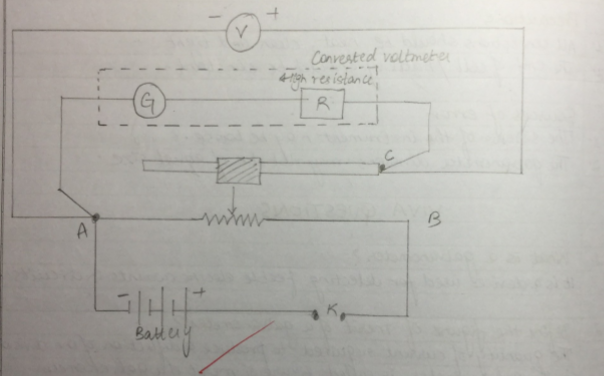# Converting the given galvanometer into a voltmeter of desired range and to verify the same

You can also get Class XII Practicals on BiologyPhysics, and Physical Education.

Aim : To convert the given galvanometer into a voltmeter of desired range and to verify the same .

Apparatus : A Weston type galvanometer , a voltmeter of 0-3 V range , a battery eliminator , two resistance boxes , the one way key , a rheostat , connecting wires .

Theory : the series resistance required for conversion ,

R=V/Ig-G

Where V is the range of conversion .

Procedure :

1. Calculate the value of series resistance R to be connected In series with the galvanometer for the given V .
2. Connect a resistance box in series with the galvanometer and take out the plug of R . The galvanometer is now ready for use as voltmeter of range V volts .
3. For verification , make the connection as shown in diagram . A and B are the fixed terminals and C is the variables terminals .
4. Take out the plugs of calculated R from the resistance box . Insert a key K and adjust the movable contact of the rheostat so that deflection in the galvanometer becomes maximum .
5. Note the readings of voltmeter and galvanometer . Convert the galvanometer reading into volts.
6. Find the difference , if any between the readings of voltmeter and galvanometer . The difference gives the error .
7. Move the variable contact C and take five observation covering whole range of voltmeter .

Result

As the difference in actual and measured value of potential difference is very small , the conversion is perfect .

Precaution

1. Length of the shunt wire should be neither too short nor too long .

2. The voltmeter used for verification should preferable be of the same range as the range of conversion .

3. Value of required series resistance should be calculated accordingly .

Sources of error

The connection may be loose .

The emf of the cell or battery may be variable .

Circuit diagram :-Observation

Resistance of given galvanometer (G) 60 ohm

No of division (n)=10

Current (Jg) =nk=0.00028A

Range of conversion = V

=0-3 V

Resistance to be placed in series = Rs

Rs =V/Ig-G

Rs =3/0.00028 -60.7

Rs = 10653.5 Ohm

Verification

V1 =3V

V2=2.8 V

% error = 3-2.8/3 x 100

=0.2/3 x 100

=6.67 %

Viva question

Why is a Galvanometer not suitable to work as an ammeter .

A galvanometer has more resistance and less current . carrying capacity from those required in ammeter . It will damage if large current flows through it .

What is a shunt ?

A small resistance connected in parallel with a galvanometer is called shunt .

Why is a galvanometer not suitable to work as voltmeter ?

A galvanometer has less resistance and more current carrying capacity from those required by a voltmeter .

How is a galvanometer converted into a voltmeter ?

A galvanometer is converted into a voltmeter by connecting a high resistance in series with the galvanometer coil.

What happens when a voltmeter is converted in series in a circuit ?

The voltmeter cannot measure the potential difference in the circuit because overall resistance of circuit increases .

You can also get Class XII Practicals on BiologyPhysics, and Physical Education.

## 13 Replies to “Converting the given galvanometer into a voltmeter of desired range and to verify the same”

1.student says:

Where is observation table?

2.Aryan says:

Please give an observation table also

3.Sangam says:

Show the observation table

1.Kyu btau says:

Bna le

4.Cialis generic says:

Hmm is anyone else encountering problems with the images on this blog loading? I’m trying to figure out if its a problem on my end or if it’s the blog. Any suggestions would be greatly appreciated.

5.hassan says:

thank for your contributions but please can you show us the observation

6.Akshay Patel AK says:

7.Ritesh akhauri says:

Where the fuck is observation table????

8.Tu madarchod says:

Observation table kidhar hai

9.Avni says:

I want conversion of galvanometer into an ammeter n it’s observation table…

10.Deep lovedeep says:

Plz show observation table

11.Tu mere gaand ka kida says:

Teri maa ka observation table kha h be

12.Priyansh Rajput (N.P.C) says:

You should also mention the vale of figure of merit i.e.,(k)